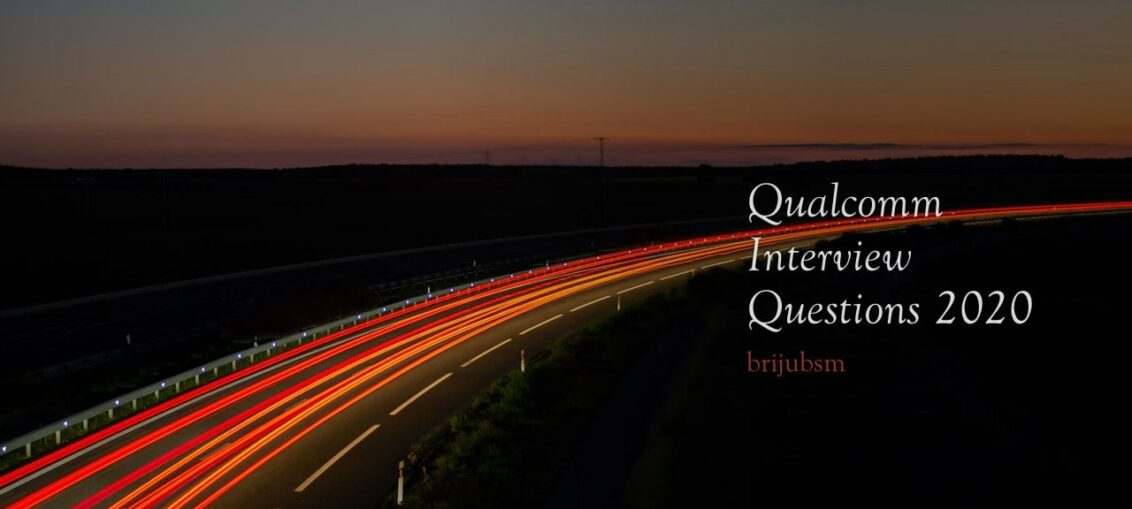You are here

# Qualcomm Interview Experience 2020This interview experience is just to guide the students about how the process happens and how the preparation level should be. No guarantee of the questions that will appear again in the exam. Do read it and understand the process. The Written exam was conducted by hirepro.

Two profiles were there Software and Hardware Engineer. You can choose between Electronics, Communication, and or Software. Each profile has three sections with each profile having 20 questions and a time limit of 30 minutes. After 30 minutes that section will be disabled.

First Section: Aptitude,

Second Section: C MCQ’s (Multiple Choice Questions),

Third Section: We can choose between any one of Electronics, Communication, or software.

## Qualcomm Aptitude

A seller sells 200 products a month for 100 each. If he increases rs.2 on each, he can sell 2 less. What will be the total money he can get if he sells Z products.

A person spends 15% on X,60% on Y,10% on Z.After all expenses, he is left with 2000. What are his expenses on X+Y?

Allegation problem mixing 80ltrs water in 180lts of wine 4 times. what is the winning quantity at the end?

Work and time problem was there.

A Person moves 100 meters straight and 50mts right, I don’t remember the complete question.

Two paragraphs and four questions on each.

Suggestion or Advice: Go through all questions once and then proceed. Because one paragraph is difficult and it takes more time.

## Qualcomm C Multiple Choice Questions

There were some questions on pointers of a two-dimensional array, constructors, methods, some compile error questions are not much tough.

8085 processor parity flag, conversion of some decimal number to binary, binary to Hexadecimal, and decimal.

Some code is given on Armstrong number, HCF and LCM code have given p+r'(q+s) is equivalent to, The full subtractor truth table, Floating-point representation.

## Qualcomm Technical

I chose the Electronics profile and the questions were easy but you should be a little fast.

1. J-K flip-flop with K inverted output Q is (the answer was JQ’ + KQ)

2. Mod-5 with JK flip-flop was given. and asked Ja. (the answer was C’)

3. Given the certain frequency with R=2.1 kOhm for F = 1.2 kHz what should be R.

4. Some questions on diffusion length given mobility and diffusion time. (based on the formula L = (D*t)^0.5)
Some problems with given electron conductivity and mobility and find hole concentration at 300K (use the formula P*n = ni2).

5. The Zener diode with V=6v at 25-degree Celcius and temperature dependence of 0.001% per degree Celcius. What is V at 125c( 6.01V)?

6. The voltage 220v at the primary end of the center-tapped transformer. What is Peak Inverse Voltage(PIV) with 1:10 (N1: N2) (the answer was 2Vm).

7. The number of 8kB chips for 256KB something like this…

8. Given Ioh, Iol, Iil, Iih currents. find the Fanout of the circuit. (the answer was min(Ioh/iih, Iol/Iil)).

9. Some PROM (Programmable read-only memory) design.

10. Some digital system design questions.

Frequency of oscillator(given some circuit).
Two input NOR gates i.e. NOR2 gate with Vtn=2Vtp and Kp=2Kn what is the threshold voltage?

Some questions related to CMOS (Complementary metal-oxide-semiconductor) design.

Also, go through,